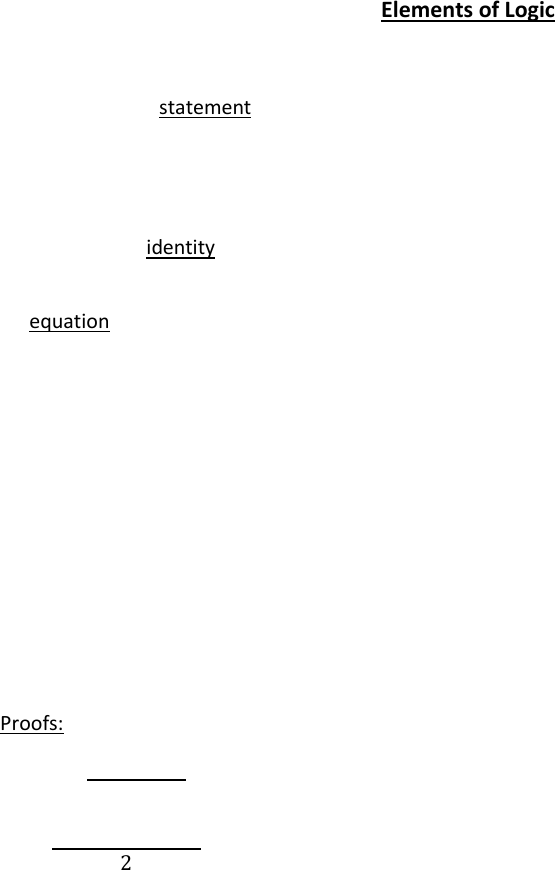# MA100 Lecture Notes - Contraposition, Prime Number, If And Only If

28 views3 pages
School
WLU
Department
Mathematics
Course
MA100
Professor
Page:
of 3Elements of Logic
In mathematics a statement is a declarative sentence that can be true or false but not both.
Ex. 7 is a prime number true
Ex. (-3, 4] εŽ false
A mathematical identity is an equality relation that is always true for all values of the variable in the
relation. Ex. (x+3)2 = x2 + 6x + 9 Ex. Sin2θ + cos2θ = 1
An equation is an equality relation depending on some variables for which we are asked to find all
values that make the statement true.
Ex. Solve x(x+3) 3(x+2) = 0
Clearly not an identity since for x=0 -6=0
X2+3x-3x-6=0
X2=6
X=±√6 – you can always check by substituting.
Solution: {-√6, √6}
(read (2) what is mathematics? Proof and solutions)
Proofs: 1) identities LS=RS
Ex. Prove 
     
   
  
    
     

2) hypothesis/conclusion
Ex. “I think therefore I am” “if a triangle is equilateral then all interior angles are 60⁰
Ex. Prove that if m and n are even numbers then m+n is also even
Proof: givens: m, n are even
m=2s, n=2t s,tεŽ
m+n= 2s+2t= 2(s+t)
 
The converse of a statement is a statement obtained by switching the hypothesis with the conclusion
Ex. I think therefore I am
Converse: I am therefore I think
If a statement is true it is not always guaranteed that its converse is true.
Ex. If m+n is even it is not guaranteed that m, n are even. Counter example: 8=3+5
3) Equivalent statements
However if a statement and its converse are true then we say that the hypothesis is equivalent to the
conclusion
HC and CH
This means H is true iff C is true
Ex. It can be shown that if a triangle has all 600 angles then it is equilateral.
Equilateral <==> all 600 angles
Note, when proving a theorem involving “iff”, you have to prove the statement both ways.
Ex. Prove that x2+y2= 0 iff x=0 and y=0
() given x2+y2=0, x,y≥0 x2=0, y2=0 x=0, y=0\
() given x=0, y=0 x2+y2=02+02=0
4) contrapositive
If we have a statement H then the contrapositive statement is: negation of C negation of H
Ex. If a triangle is equilateral then all its interior angles are 600
Contrapositive: if not all interior angles are 600 then a triangle is not equilateral.
A statement and its contrapositive are logically equivalent. Thus if we want we can prove the
contrapositive of a statement rather than proving the original statement.

H: 3n+7 is odd C: n is even
Contrapositive
Not C: n is odd Not H: 3n+7 is even

3n+7= 3(2k+1)+7
= 6k+3+7
=6k+10
=2(3k+5)
   
Since we have proved the contrapositive we have proved the original

## Document Summary

In mathematics a statement is a declarative sentence that can be true or false but not both. A mathematical identity is an equality relation that is always true for all values of the variable in the relation. Ex. (x+3)2 = x2 + 6x + 9 ex. An equation is an equality relation depending on some variables for which we are asked to find all values that make the statement true. Clearly not an identity since for x=0 -6=0. X= 6 you can always check by substituting. I think therefore i am if a triangle is equilateral then all interior angles are 60 . Prove that if m and n are even numbers then m+n is also even. The converse of a statement is a statement obtained by switching the hypothesis with the conclusion. If a statement is true it is not always guaranteed that its converse is true.